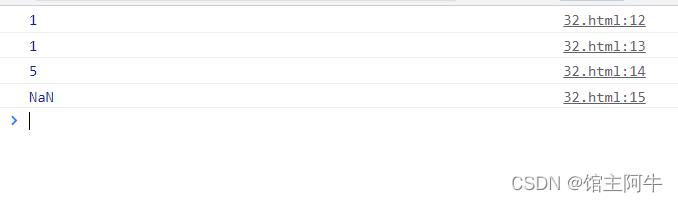﻿ javascript内置对象Math案例总结分析 - 自由资讯

# javascript内置对象Math案例总结分析

##### 目录
• Math概述
• Math中常用函数的用法
• 1.绝对值方法
• 2.三个取整方法
• 3.求最大值/最小值
• 4.随机数
• 案例
• 求两个数之间的随机整数的小算法（重要）
• 随机点名
• 结语

## Math概述

Math 对象不是构造函数，它具有数学常数和函数的属性和方法。跟数学相关的运算（求绝对值，取整、最大值等）可以使用 Math 中的成员。

## Math中常用函数的用法

```Math.PI //圆周率
Math.floor () //向下取整
Math.ceil () //向上取整
Math.round () //四舍五入就近取整 注意﹣3.5 结果是-3
Math.abs () //绝对值
Math.max ()/Math.min() //求最大值和最小值
Math.random() //返回一个随机的小数 0=<x<1(这个方法里面不跟参数)```

### 1.绝对值方法

``` //1.绝对值方法
console.log(Math.abs(1)); // 1
console.log(Math.abs(-1));  //1
console.log(Math.abs('-5')); //5  会隐式转换，将数字字符串转换为数字，然后取绝对值
console.log(Math.abs('aniu')); // NaN
```### 2.三个取整方法

```//2.三个取整方法
console.log(Math.floor(1.1)); //1
console.log(Math.floor(1.9)); //1
console.log(Math.floor(-1.1)); //-2
console.log(Math.ceil(1.1));  // 2
console.log(Math.ceil(1.9)); //2
console.log(Math.ceil(-1.9)); //-1
console.log(Math.round(1.5)); //2 四舍五入 .5这个特殊，是往大了取
console.log(Math.round(-1.5)); // -1  往大了取
console.log(Math.round(-1.2));  // -1
```### 3.求最大值/最小值

```//3.求最大值/最小值
console.log(Math.max(1,5,78,46));
console.log(Math.min(1,5,78,46));```### 4.随机数

``` //4.随机数
console.log(Math.random());
```## 案例

### 求两个数之间的随机整数的小算法（重要）

求两个数之间的随机整数 并且包含这两个数:

```//核心算法
Math.floor(Math.random()*(max-min)) + min;```
```function getRandom(min,max){
return Math.floor(Math.random()*(max-min)) + min;
}
console.log(getRandom(1,7));
```### 随机点名

```//随机点名
var arr = ['阿牛','梦梦','小鸣人','winter','小何','WA','贱神','扎哇']  //太多啦，就写这些举例啦
console.log(arr);
console.log('阿牛爱你们 ```
上一篇：javascript数组中的findIndex方法
下一篇：没有了
网友评论
相关栏目
﻿